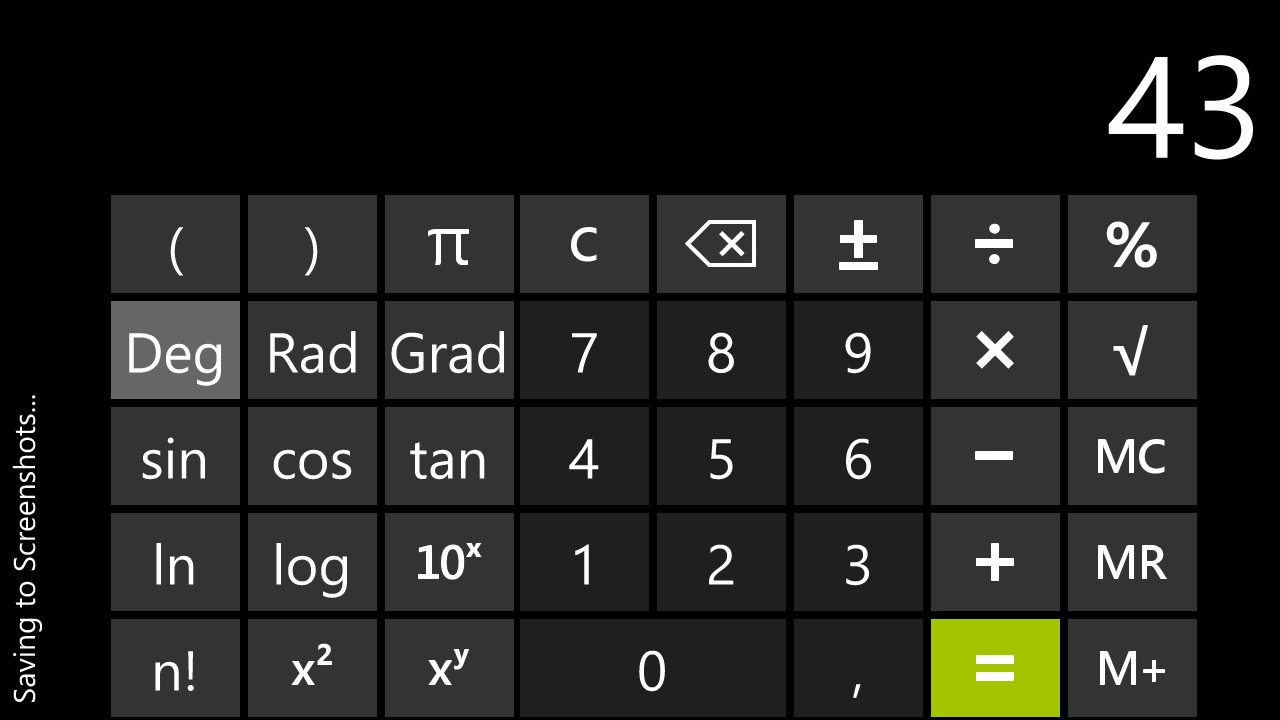Select Page

Nowadays, one should assume, that a calculator knows all simple mathematical rules no matter how you hold it in your hand. However, that is not true for the calculator of Windows Phone 8 (WP8).

Imagine the following example: 3 + 5 x 8 = ??

The clever user knows, that you have to multiply 5 and 8 before you can add 3. Thus, the correct answer to the example is 43. Take your WP8 calculator in landscape mode, try the example and you you will end up with the result depicted in the following WP8 screenshot:Now, try the same, but, before that, turn your phone in a vertical position. Of course, the result is the same… NOOOO!!! It is not:64??? 3 +5 x 8 = 64??? Yes, if you ignore one simple mathematical rule: It calculates 3+5 = 8 and then multiply 8 to the sum.

Bug or feature?

We assume that this is a feature: The calculator in vertical mode imitates the old, simple commercial calculator. Now we know… 😉